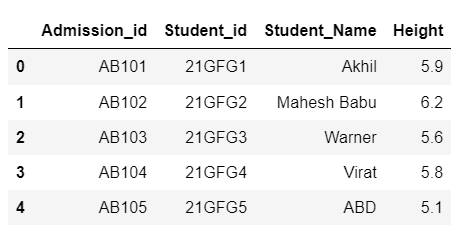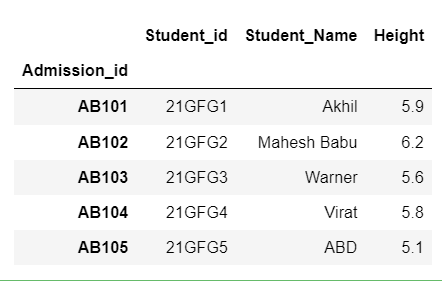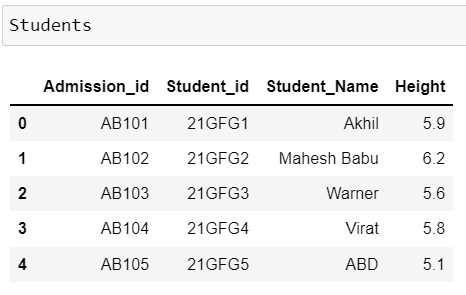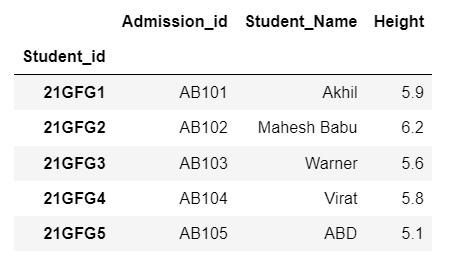GFG App
Open AppBrowser
Continue

# How to Change Index Values in Pandas?

Index is used to uniquely identify a row in Pandas DataFrame. It is nothing but a label to a row. If we didn’t specify index values to the DataFrame while creation then it will take default values i.e. numbers starting from 0 to n-1 where n indicates a number of rows.

Let’s create a dataframe

Example:

## Python3

 `# import necessary packages` `import` `pandas as pd`   `# create a dataframe` `Students ``=` `pd.DataFrame({``'Admission_id'``: [``'AB101'``, ``'AB102'``, ``'AB103'``,` `                                          ``'AB104'``, ``'AB105'``],` `                         ``'Student_id'``: [``'21GFG1'``, ``'21GFG2'``, ``'21GFG3'``, ` `                                        ``'21GFG4'``, ``'21GFG5'``],` `                         ``'Student_Name'``: [``'Akhil'``, ``'Mahesh Babu'``, ``'Warner'``,` `                                          ``'Virat'``, ``'ABD'``],` `                         ``'Height'``: [``5.9``, ``6.2``, ``5.6``, ``5.8``, ``5.10``]})` `# display dataframe` `Students`

Output:## Method  1 : Using set_index()

To change the index values we need to use the set_index method which is available in pandas allows specifying the indexes.

Syntax

DataFrameName.set_index(“column_name_to_setas_Index”,inplace=True/False)

where,

• inplace parameter accepts True or False, which specifies that change in index is permanent or temporary.
• True indicates that change is Permanent.
• False indicates that the change is Temporary.

Example 1:

Changing the index temporarily by specifying inplace=False (or) we can make it without specifying inplace parameter because by default the inplace value is false.

## Python3

 `# import necessary packages` `import` `pandas as pd`   `# create a dataframe` `Students ``=` `pd.DataFrame({``'Admission_id'``: [``'AB101'``, ``'AB102'``, ``'AB103'``, ` `                                          ``'AB104'``, ``'AB105'``],` `                         ``'Student_id'``: [``'21GFG1'``, ``'21GFG2'``, ``'21GFG3'``,` `                                        ``'21GFG4'``, ``'21GFG5'``],` `                         ``'Student_Name'``: [``'Akhil'``, ``'Mahesh Babu'``, ``'Warner'``,` `                                          ``'Virat'``, ``'ABD'``],` `                         ``'Height'``: [``5.9``, ``6.2``, ``5.6``, ``5.8``, ``5.10``]})`   `# setting admission id as index but temporarily` `Students.set_index(``"Admission_id"``)`

Output:But when we displayed the data in DataFrame but it still remains as previous because the operation performed was not saved as it is a temporary operation.Explanation– As we didn’t specify inplace parameter in set_index method, by default it is taken as false and considered as a temporary operation.

Example 2 :

Changing the index permanently by specifying inplace=True in set_index method.

Example:

## Python3

 `# import necessary packages` `import` `pandas as pd`   `# create a dataframe` `Students ``=` `pd.DataFrame({``'Admission_id'``: [``'AB101'``, ``'AB102'``, ``'AB103'``, ` `                                          ``'AB104'``, ``'AB105'``],` `                         ``'Student_id'``: [``'21GFG1'``, ``'21GFG2'``, ``'21GFG3'``, ` `                                        ``'21GFG4'``, ``'21GFG5'``],` `                         ``'Student_Name'``: [``'Akhil'``, ``'Mahesh Babu'``, ``'Warner'``,` `                                          ``'Virat'``, ``'ABD'``],` `                         ``'Height'``: [``5.9``, ``6.2``, ``5.6``, ``5.8``, ``5.10``]})`   `# setting student id as index but permanently` `Students.set_index(``"Student_id"``, inplace``=``True``)`   `# display dataframe` `Students`

Output:Example 3  :

When we want to retrieve only particular columns instead of all columns follow the below code

## Python3

 `# import necessary packages` `import` `pandas as pd`   `# create a dataframe` `Students ``=` `pd.DataFrame({``'Admission_id'``: [``'AB101'``, ``'AB102'``, ``'AB103'``,` `                                          ``'AB104'``, ``'AB105'``],` `                         ``'Student_id'``: [``'21GFG1'``, ``'21GFG2'``, ``'21GFG3'``, ` `                                        ``'21GFG4'``, ``'21GFG5'``],` `                         ``'Student_Name'``: [``'Akhil'``, ``'Mahesh Babu'``, ``'Warner'``,` `                                          ``'Virat'``, ``'ABD'``],` `                         ``'Height'``: [``5.9``, ``6.2``, ``5.6``, ``5.8``, ``5.10``]})`   `# setting student id as index but permanently` `Students.set_index(``"Student_id"``, inplace``=``True``)`   `# display dataframe with required columns` `Students[[``"Student_Name"``, ``"Height"``]]`

OutputMy Personal Notes arrow_drop_up